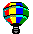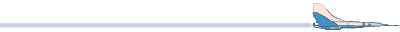# From the 1982 ARRL antenna book. 1.  cos(D) = (sin(A) * sin(B)) + (cos(A) * cos(B) * cos(L)) 2.  cos(C) = (sin(B) - (sin(A) * cos(D))) / (cos(A) * sin(D)) WHERE: A = YOUR latitude in degrees. B = latitude of the other location in degrees. L = YOUR longitude minus that of the other location. (Algebraic difference.) D = Distance along path in degrees of arc. C = True bearing from north if the value for sin(L) is positive.  If sin(L) is negative, true bearing is 360 - C. To convert degrees of arc to statute miles, multiply by 69.041. To convert degrees of arc to kilometers, multiply by 111.111.CAN'T FIND IT? SEARCH THE WEB OR THE AC6V WEBSITEAC6V BRAG TAPELast Upated: March 26, 2006